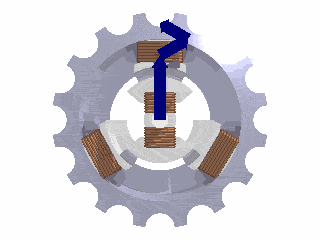Home » Posts tagged 'Alternating current'

# Tag Archives: Alternating current

## Single phase, two phase, three phase and multiphase electric generators – which one is more efficient?

I started referring to the above issue when one students asked, “Why do we use three phase generation of power and not two phase or four phase?”

Three-phase has properties that make it very desirable in electric power systems:

The phase currents tend to cancel out one another, summing to zero in the case of a linear balanced load. This makes it possible to reduce the size of the neutral conductor because it carries little to no current; all the phase conductors carry the same current and so can be the same size, for a balanced load.Vector sum of the magnetic field vectors of the stator coils produces a single rotating vector of resulting rotating magnetic field. (Photo credit: Wikipedia)

Power transfer into a linear balanced load is constant, which helps to reduce generator and motor vibrations.

Three-phase systems can produce a rotating magnetic field with a specified direction and constant magnitude, which simplifies the design of electric motors.

Refer the following links for more …

http://en.wikipedia.org/wiki/Two-phase_electric_power

http://en.wikipedia.org/wiki/Polyphase_system

http://en.wikipedia.org/wiki/Three-phase_electric_power## Questions from Alternating current

1.      Derive an expression for the average power in an ac circuit.
2.      Distinguish between resistance, reactance and impedance.
3.      Draw the phasor diagram showing voltage and current in LCR series circuit and derive an expression for the impedance4.      Deduce the phase relationship between current and voltage in an ac circuit containing inductor only
5.      A series LCR circuit with L = 0.12 H, C = 4.8 x 10 7 f and R = 23 ohms is connected to a variable frequency supply.  At What frequency is the current maximum?
9.      A sinusoidal voltage V=200 sin 314t is applied to a resistor of 10 ohms resistance. Calculate (i) rms value of the voltage, (ii) rms value of the current and (iii) power dissipated as heat in watts.

(Posted by Sayantika Nath)### Visitors So Far @ AskPhysics

• 2,166,832 hits

### Subscribe to Blog via EmailAsk Physics